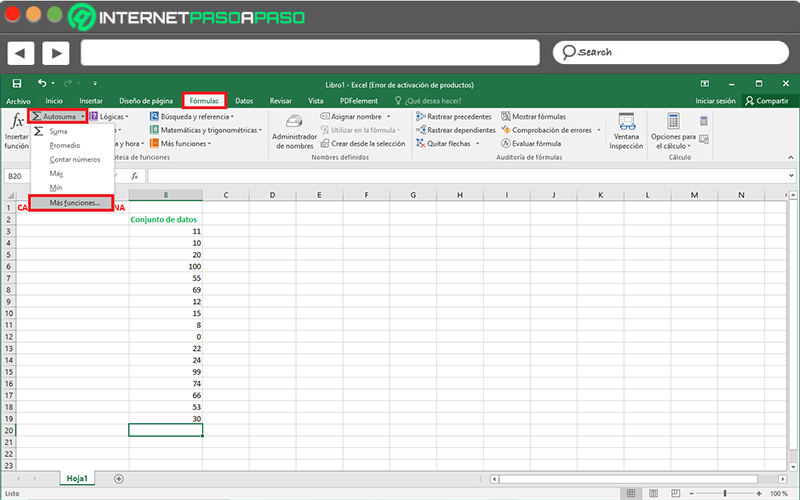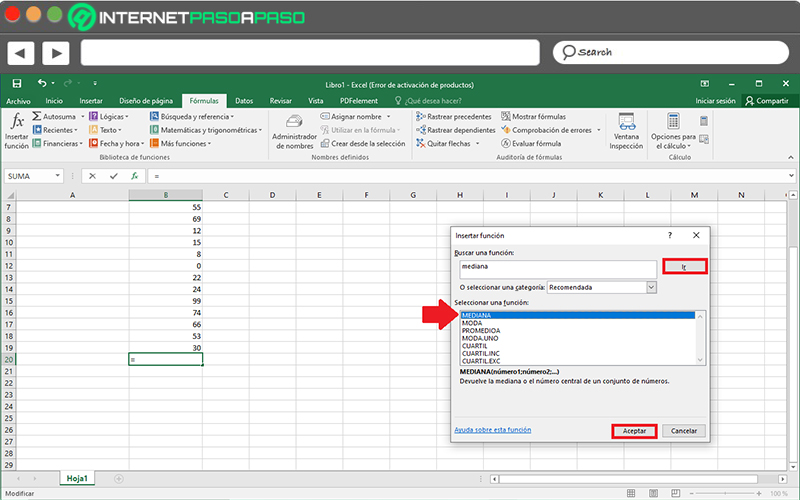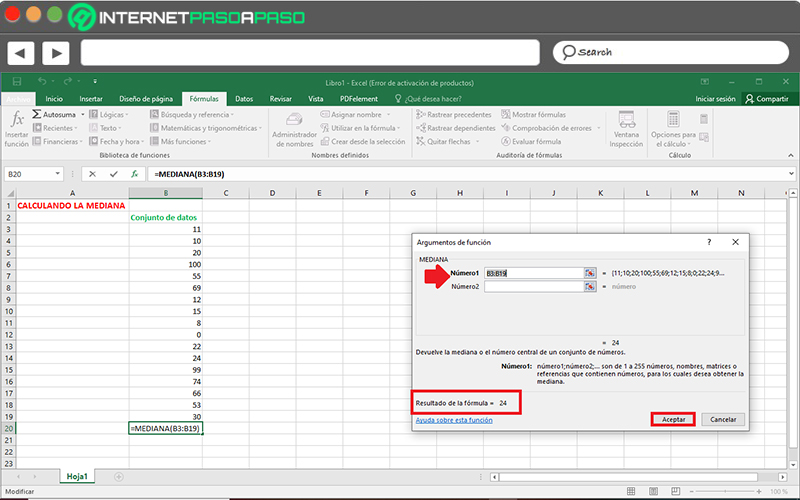Calculate Median in Excel  Step by Step Guide ▷ 2020

It is no secret to anyone that, by default, Excel has been designated as the leading spreadsheet software used to carry out mathematical, statistical and financial tasks. Therefore, it is an essential tool for many students and professionals today.

In this sense, this program belonging to the Microsoft Office suite, it is ideal for perform all kinds of numerical and mathematical operations, in a very simple and effective way. Since, it has endless functions that the users themselves can customize.

Thus, at the statistical level, Microsoft Excel has been classified as a very useful tool for carrying out different investigations. Reason for which, allows you to quickly obtain the median of a data set and, in this post, we will teach you how can you calculate it.

What is the median and what is this value for in statistics?

Basically, in the field of statistics, the median of a group of digits consists of that number or value that represents the average position of said set. That is, it is the central number of certain values ​​that are organized by size and therefore you have just half of the numbers with a higher value and half of the numbers with a lower value.

For its part, it is a type of data that, in general, is denoted as “Me” and although it presents some similarity with the handling of the arithmetic mean, the truth is that the two terms should not be confused. Well, while the latter is an average obtained from the relationship of all the numbers that make up a group, the median is simply the intermediate value of a data set.

Now, regarding the specific use that this value has in statistics, we distinguish that its main purpose is highlight the core value of a hierarchical order of numbers. Thus, its application is based on specify the figure that is in the middle of a group of digits used for any study. Thus, for example, it is used to return the central tendency in the case of cross-sectional numerical distributions.

What should we take into account when trying to obtain a statistical median?Although, by means of Microsoft Excel, the process to calculate the median of a set of values ​​can be completely quick and easy to carry out, in reality also it is worth taking into account certain general aspects when measuring a statistical median.

In this sense, to find out the central tendency of any group of numbers manually, it is important to understand that, these have to be ordered according to their size. Added to this, if the total number of terms it’s even, the two values ​​in the middle must be added and divided by 2. On the other hand, if the number of digits it’s odd, the median will be that central value.

On the other hand, when this type of statistical operation is carried out making use of Excel, the program takes into consideration certain parameters to be able to let you know the specific value.

Then, We mention what these aspects are:

• In the event that the total of values ​​that make up the set to be studied is even, the median will be obtained from the average of the central numbers.
• Regarding the arguments, they can be both numbers and also names, references or arrays that have some numerical data.
• If it is a reference or matrix argument and this contains text, empty cells or logical values, Microsoft Excel will ignore such information.
• Yes, within the table to study to calculate the median there are cells with the value zero, these will also be included during the calculation.
• Without any exception, logical values ​​and textual representations of data written in the list of arguments under study are always taken into account, directly.
• It is necessary to know that, if the arguments are text or error values ​​that cannot be translated into numbers by the same software, errors will automatically originate in the final result.

Learn step by step how to calculate the median in your Excel documents

Finally, to know how to use this spreadsheet program correctly when perform operations such as computing the median of a data set, it is important to detail the process to be carried out step by step. So then We proceed to carry out this explanation of great interest to many students and professionals from different areas:

• First of all, proceed to open a blank Excel workbook or spreadsheet to add the group of numbers to which you have to calculate their statistical median.
• Once you include these digits as a list, click inside any empty cell in the document (In this case, we place the cursor in the cell below the last number in the set).
• Followed by this, go to the program options bar (at the top) and click on the tab that says “Formulas”. There, in the Function Library group, press the “AutoSum” option and select “More functions”.• Later, in the box that says “Find a function”, you just have to choose write the term “median” and click “Go”. Once the software locates this function, select it to click on the “OK” button.• Then, you must insert the identification of the first and last cell corresponding to the list of your group of numbers. But in general, if you do this whole procedure with the cursor located in the cell after the last digit, the program will choose the listing correctly on its own.
• When you check that the numbers of these cells are correct in the table “number 1”, click on the button “To accept” and immediately the result will be displayed in the indicated box of the spreadsheet (which, you can also view from this last dialog).In addition to this procedure, there is also a slightly more practical way to calculate the median of a group of data from Excel. So, the method is based on proceeding directly from the “Home” tab and in the Modify group, select the option that indicates “Autosum”, in order to carry out the same process detailed above.

If you have any questions, leave them in the comments, we will answer you as soon as possible, and it will also be of great help to more members of the community. Thank you! 😉

Rate this post